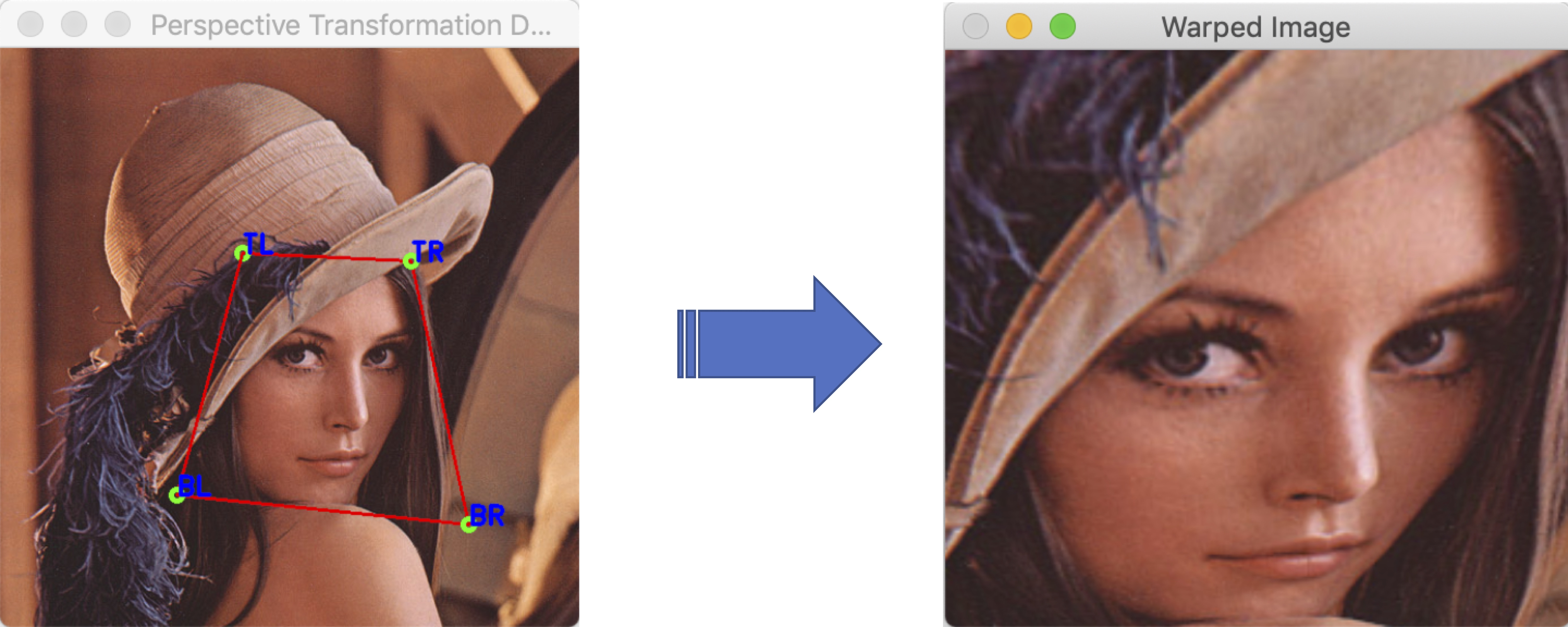# OpenCV C++学习笔记(4): 4点投影变换vector< Point2f> roi_corners;
vector< Point2f> dst_corners(4);


Mat H = findHomography(roi_corners, dst_corners);


si[dst_x, dst_y, 1] = H * [roi_x, roi_y, 1]

• dst_x = (H00 * x + H01 * y + H02 * 1) / (H20 * x + H21 * y + H21 * 1);
• dst_y = (H10 * x + H11 * y + H12 * 1) / (H20 * x + H21 * y + H21 * 1)

Mat warped_image;
warpPerspective(
original_image,
warped_image,
H,
warped_image_size);